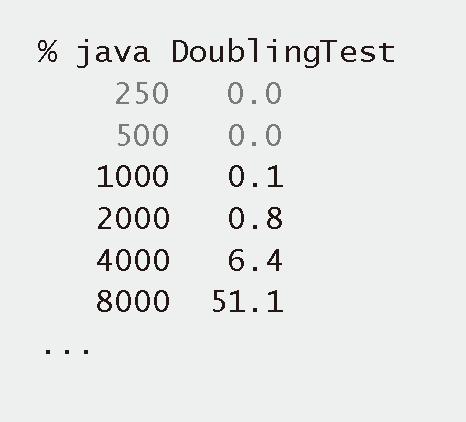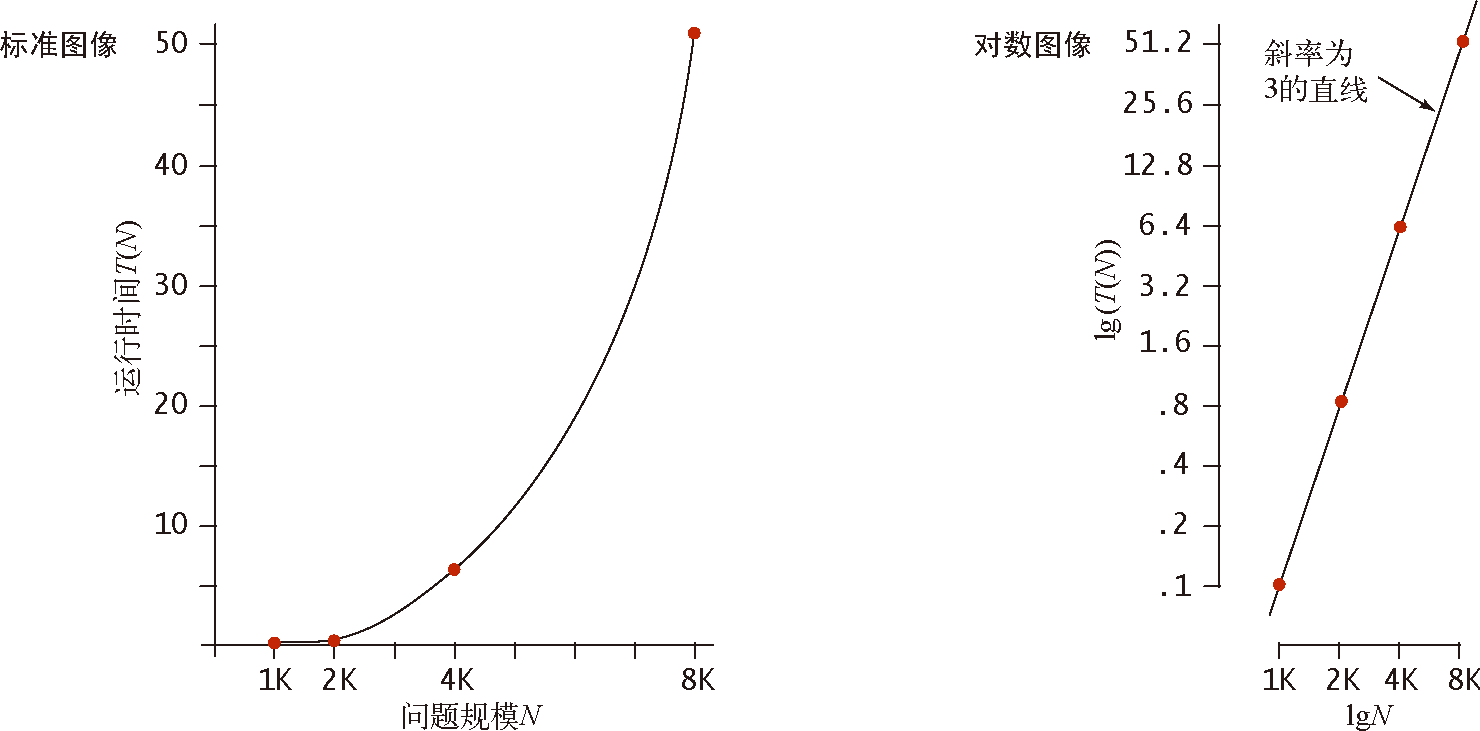# 算法（4th ed）(150)：基础——算法分析 6.2.3(观察：实验数据的分析）

DoublingTest 是Stopwatch 的一个更加复杂的用例，并能够为 ThreeSum 产生实验数据。它会生成一系列随机输入数组，在每一步中将数组长度加倍，并打印出ThreeSum.count() 处理每种输入规模所需的运行时间。这些实验显然是可重现的——你也可以在自己的计算机上运行它们，多少次都行。在运行 DoublingTest 时，你会发现自己进入了一个“预测—验证”的循环：它会快速打印出几行数据，但随即慢了下来。每当它打印出一行结果时，你都会开始琢磨它还需要多久才能打出下一行。当然，因为大家使用的计算机不同，你得到的实际运行时间很可能和我们的计算机得到的不一样。事实上，如果你的计算机比我们的快一倍，你所得到的运行时间应该大致是我们所得到的一半。由此我们马上可以得出一条有说服力的猜想：程序在不同的计算机上的运行时间之比通常是一个常数。尽管如此，你还是会提出更详细的问题：作为问题规模的一个函数，我的程序的运行时间是多久？为了帮助你回答这个问题，我们来将数据绘制成图表。图 1.4.2 就是产生结果，使用的分别是标准比例尺和对数比例尺。其中 $x$ 轴表示 $N$$y$ 轴表示程序的运行时间 $T(N)$。由对数的图像我们立即可以得到一个关于运行时间的猜想——因为数据和斜率为 3 的直线完全吻合。该直线的公式为（其中 a 为常数）：

$\lg(T(N))=3\lg N+\lg a$

$T(N)=aN^3$

$T(N)=9.98\times10^{-11}N^3$

public class DoublingTest{   public static double timeTrial(int N)   {  // 为处理 N 个随机的六位整数的 ThreeSum.count() 计时      int MAX = 1000000;      int[] a = new int[N];      for (int i = 0; i < N; i++)         a[i] = StdRandom.uniform(-MAX, MAX);      Stopwatch timer = new Stopwatch();      int cnt = ThreeSum.count(a);      return timer.elapsedTime();   }   public static void main(String[] args)   {  // 打印运行时间的表格      for (int N = 250; true; N += N)      {  // 打印问题规模为 N 时程序的用时         double time = timeTrial(N);         StdOut.printf("%7d %5.1f\n", N, time);      }   }}